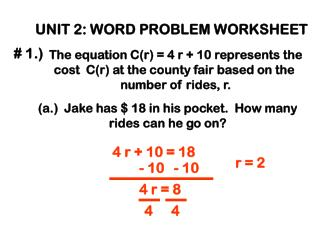DownloadDownload Presentation# 1.)

# # 1.)

Download Presentation## # 1.)

- - - - - - - - - - - - - - - - - - - - - - - - - - - E N D - - - - - - - - - - - - - - - - - - - - - - - - - - -
##### Presentation Transcript

1. UNIT 2: WORD PROBLEM WORKSHEET # 1.) The equation C(r) = 4 r + 10 represents the cost C(r) at the county fair based on the number of rides, r. (a.) Jake has \$ 18 in his pocket. How many rides can he go on? 4 r + 10 = 18 r = 2 - 10 - 10 4 r = 8 4 4

2. UNIT 2: WORD PROBLEM WORKSHEET # 1.) The equation C(r) = 4 r + 10 represents the cost C(r) at the county fair based on the number of rides, r. (b.) If Jake wants to ride 10 rides, how much money does he have to borrow from his friends? 4 (10) + 10 = C (r) 40 + 10 = C (r) 50 – 18 = amt. to borrow 50 = C (r) 32 = amt. to borrow

3. UNIT 2: WORD PROBLEM WORKSHEET # 2.) The cost of a pizza is based on the number of toppings plus \$ 8. The cost of a 5-topping pizza is \$ 14.25. How much would a 3-topping pizza cost? Let x = cost of one topping 5 x + 8 = 14.25 x = 1.25 = cost of one topping - 8 - 8 5 x = 6.25 3 (1.25) + 8 = 3.75 + 8 = 11.75 = cost of 3-topping pizza 5 5

4. 4 5 UNIT 2: WORD PROBLEM WORKSHEET # 3.) A case of 20 soft drinks is \$ 12.00. How much does James have to sell each soft drink for to make a profit of \$ 4 per case? Case would sell for \$ 16.00. x = cost of one soft drink 20 x = 16 = 20 20 Each drink would need to sell for \$ 0.80.

5. UNIT 2: WORD PROBLEM WORKSHEET # 4.) Peter is selling cookies. He sells each cookie for \$ 0.75. He had to spend \$ 15 on supplies. How many cookies does he need to sell to earn \$ 10? x = number of cookies x = 33.33 cookies .75 x = 15 + 10 100 • .75 x = 25 • 100 He would need to sell at least 34 cookies. 75 x = 2500 75 75

6. UNIT 2: WORD PROBLEM WORKSHEET # 5.) Joseph’s dad is three less than twice Joseph’s age. Together, their ages are 63. How old is Joseph? Joseph’s age: x 22 Dad’s age: 2 x – 3 x + 2 x – 3 = 63 3 x – 3 = 63 + 3 + 3 3 x = 66 x = 22 3 3

7. UNIT 2: WORD PROBLEM WORKSHEET # 6.) Jack weighs 158 pounds. This is 2 less than ¾ of his father’s weight. How much does his father weigh? x = father’s weight ¾ x – 2 = 158 + 2 + 2 ¾ x = 160 x = 640/3 = 213.3 pounds

8. p2 E UNIT 2: WORD PROBLEM WORKSHEET # 7.) Solve for c: • c c • c • E = p2 E E c =

9. UNIT 2: WORD PROBLEM WORKSHEET # 8.) Mt + Tabor = Good School Solve for b:

10. UNIT 2: WORD PROBLEM WORKSHEET # 9.) A large bath tub is losing 1.5 gallons every minute. At 8:00 pm, there were 32 gallons. At what time were there 17 gallons?

11. UNIT 2: WORD PROBLEM WORKSHEET # 10.) 3 (x – 2) = 12. What is 2 x – 5?

12. UNIT 2: WORD PROBLEM WORKSHEET # 11.) Solve for x.

13. UNIT 2: WORD PROBLEM WORKSHEET # 12.) 3 (x – 2) = 5 (x + 2) + 1 Solve for x.

14. UNIT 2: WORD PROBLEM WORKSHEET # 13.) Find the mistake (if any) in the following problem: Then solve it correctly. - 2 ( x – 2) – 3 x = 10 + 5 x - 2 x + 4 – 3 x = 10 + 5 x - 5 x + 4 = 10 + 5 x 0 x + 4 = 10 0 = 6 No solution